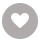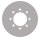《跟美国学生同步练阅读》是北美教师依据现行美国中小学教学大纲“共同核心标准”(Common Core State Standards) 编写而成，《跟美国学生同步练阅读》采用全英文方式编写，为出国留学早准备。《跟美国学生同步练阅读》是继《美国语文》、《英国语文》等经典教材之后，专门为国内准备留学的中小学生定制的全英文学习辅导读本，每一课后都有同步习题。对其他英语学习者，亦可适用。

131人正在读 | 0人评论6.2

• 读书简介
• 目录
• 累计评论(3条)
• 读书简介
• 目录
• 累计评论(3条)
【推荐语】
《跟美国学生同步练阅读》是北美教师依据现行美国中小学教学大纲“共同核心标准”(Common Core State Standards) 编写而成，《跟美国学生同步练阅读》采用全英文方式编写，为出国留学早准备。《跟美国学生同步练阅读》是继《美国语文》、《英国语文》等经典教材之后，专门为国内准备留学的中小学生定制的全英文学习辅导读本，每一课后都有同步习题。对其他英语学习者，亦可适用。

Lesson 1

Lesson 2

Lesson 3

Lesson 4

Lesson 5

Lesson 6

Lesson 7

Lesson 8

Lesson 9

Lesson 10

Lesson 11

Lesson 12

Lesson 13

Lesson 14

Lesson 15

Lesson 16

Lesson 17

Lesson 18

Lesson 19

Lesson 20

Lesson 21

Lesson 22

Lesson 23

Lesson 24

Lesson 25

Lesson 26

Lesson 27

Lesson 28

Lesson 29

Lesson 30

Lesson 31

Lesson 32

Lesson 33

Lesson 34

Lesson 35

Lesson 36

Lesson 37

Lesson 38

Lesson 39

Lesson 40

Lesson 41

Lesson 42

Lesson 43

Lesson 44

Lesson 45

Lesson 46

Lesson 47

Lesson 48

Lesson 49

Lesson 50

Lesson 51

Lesson 52

Lesson 53

Lesson 54

Lesson 55

Lesson 56

Lesson 57

Lesson 58

Lesson 59

Lesson 60

Lesson 61

Lesson 62

Lesson 63

Lesson 64

Lesson 65

01

￥59.99
02

￥15.92
03

￥19.90
04

￥27.00
05

￥54.00
06

￥3.14
07

￥12.60
08

￥0.10
09

￥11.20
10

### 栀子猫的奇幻编程之旅——21天探索信息学奥赛C++编程￥39.00

￥39.00
•关注我们

最受欢迎的阅读产品

关注我们：

•关于我们

欢迎反馈宝贵意见给我们

客服书吧：当当云阅读问答